# Dr Frost Cumulative Frequency Graphs Starter Problems involving

• Slides: 37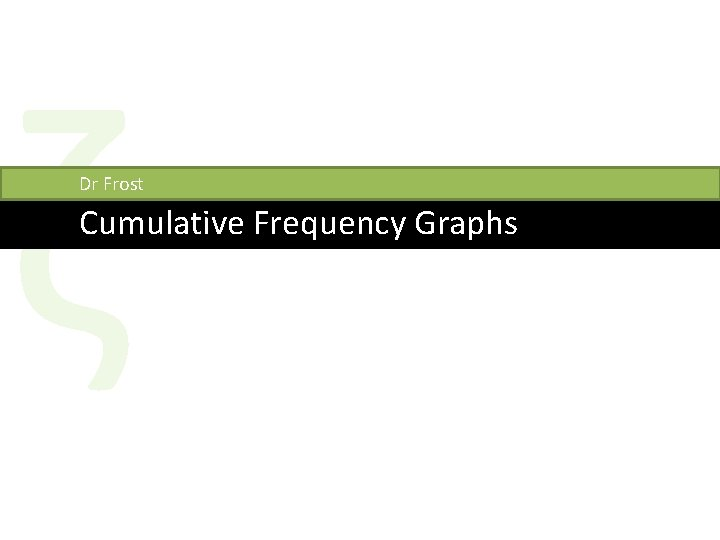ζ Dr Frost Cumulative Frequency GraphsStarter: Problems involving mean ? ? Girl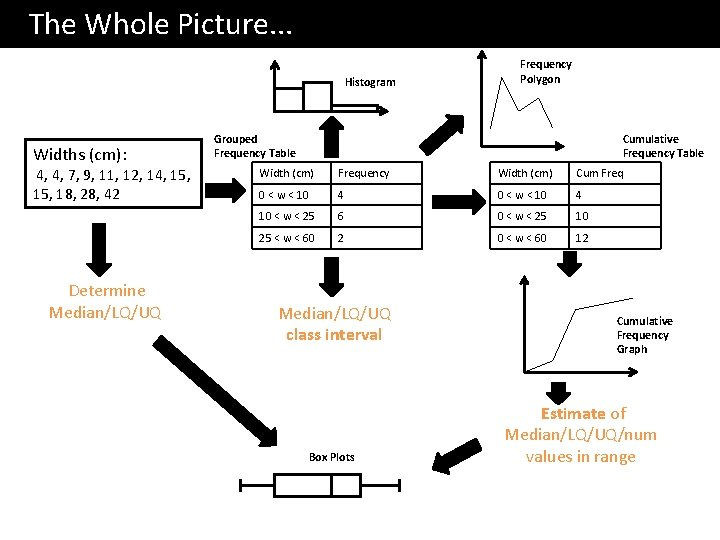The Whole Picture. . . Histogram Widths (cm): 4, 4, 7, 9, 11, 12, 14, 15, 18, 28, 42 Determine Median/LQ/UQ Frequency Polygon Cumulative Frequency Table Grouped Frequency Table Width (cm) Frequency Width (cm) Cum Freq 0 < w < 10 4 10 < w < 25 6 0 < w < 25 10 25 < w < 60 2 0 < w < 60 12 Median/LQ/UQ class interval Box Plots Cumulative Frequency Graph Estimate of Median/LQ/UQ/num values in range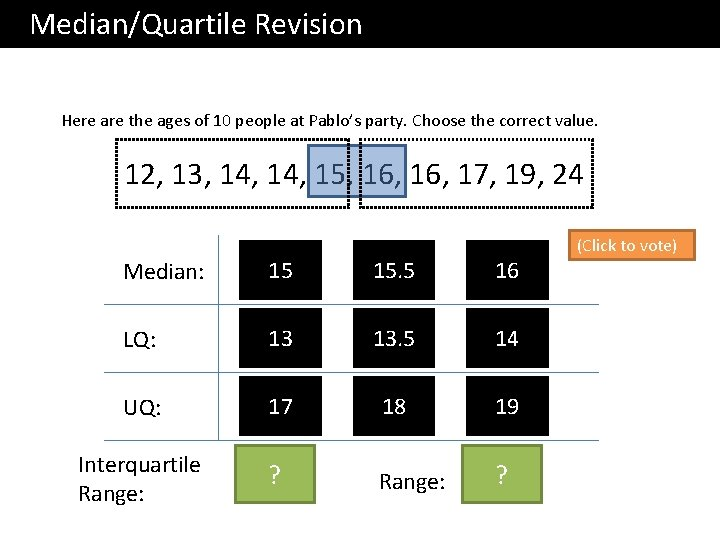Median/Quartile Revision Here are the ages of 10 people at Pablo’s party. Choose the correct value. 12, 13, 14, 15, 16, 17, 19, 24 Median: 15 15. 5 16 LQ: 13 13. 5 14 UQ: 17 18 19 Interquartile Range: 3? Range: ? 12 (Click to vote)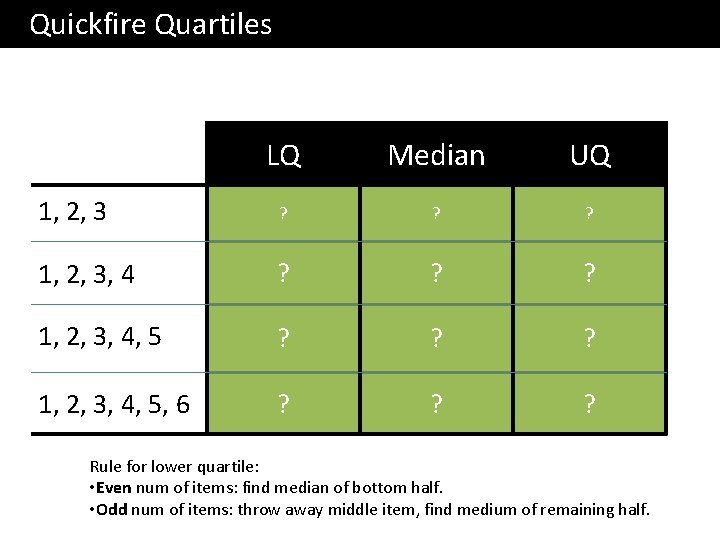Quickfire Quartiles LQ Median UQ 1? 2? 3? 1, 2, 3, 4 ? 1. 5 ? 2. 5 ? 3. 5 1, 2, 3, 4, 5 1. 5 ? 2? 4. 5 ? 2? 3. 5 ? 5? 1, 2, 3, 4, 5, 6 Rule for lower quartile: • Even num of items: find median of bottom half. • Odd num of items: throw away middle item, find medium of remaining half.What if there’s lots of items? There are 31 items, in order of value. What items should we use for the median and lower/upper quartiles? 0 1 1 2 4 5 5 6 7 8 10 14 14 15 16 17 29 31 37 38 39 40 41 43 44 LQ th item Use the 8 ? Median ? th item Use the 16 UQ ? th item Use the 24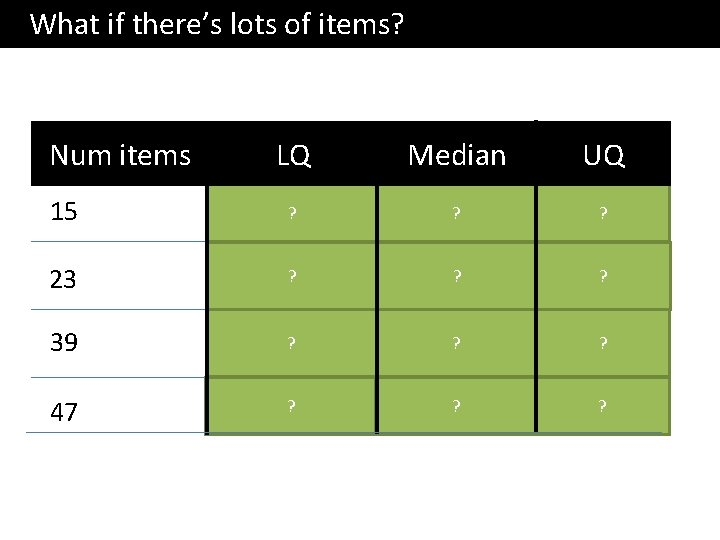What if there’s lots of items? Num items LQ Median UQ 15 4 th? 8? th 12 23 6 th? 12? th 18? th 39 10? th 20? th 30? th 47 12? th 24? th 36? thBox Plots allow us to visually represent the distribution of the data. Minimum Maximum Median Lower Quartile Upper Quartile 3 27 17 15 Sketch 22 Sketch range IQR 0 5 10 15 20 25 30 How is the IQR represented in this diagram? Sketch How is the range Sketch represented in this diagram?Box Plots Sketch a box plot to represent the given weights of cats: 5 lb, 6 lb, 7. 5 lb, 8 lb, 9 lb, 12 lb, 14 lb, 20 lb Minimum 5 ? Maximum 20 ? Median 8 ? Lower Quartile Upper Quartile 6. 75 ? 13 ? 0 4 8 12 16 20 24 Sketch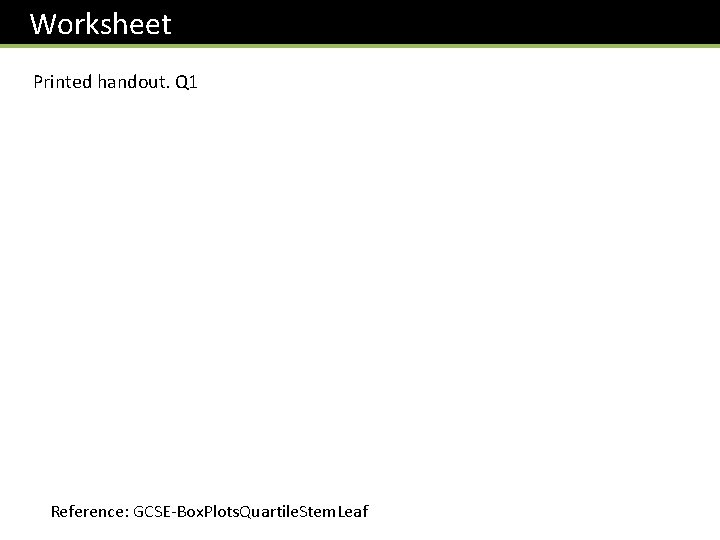Worksheet Printed handout. Q 1 Reference: GCSE-Box. Plots. Quartile. Stem. LeafBox Plots If you only had the diagram, how could we interpret the distribution of data? What we observe What we can deduce The second box is wider than the first. There is a greater spread of weights in the top ? half. This is known as positive skew. The length of the second ‘whisker’ (pun intended) is quite long. The fattest cat has a weight that is an ? extreme value, because the weight is far above the Upper Quartile. 0 4 8 12 16 20 24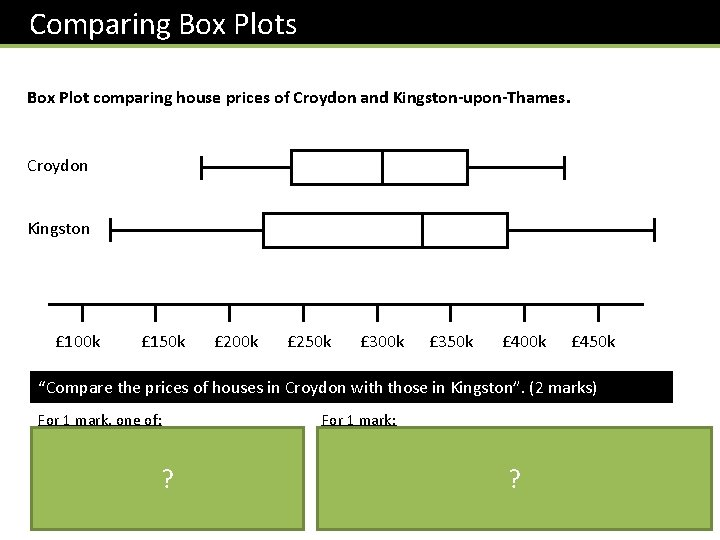Comparing Box Plots Box Plot comparing house prices of Croydon and Kingston-upon-Thames. Croydon Kingston £ 100 k £ 150 k £ 200 k £ 250 k £ 300 k £ 350 k £ 400 k £ 450 k “Compare the prices of houses in Croydon with those in Kingston”. (2 marks) For 1 mark, one of: • In interquartile range of house prices in Kingston is greater than Croydon. • The range of house prices in Kingston is greater than Croydon. ? For 1 mark: • The median house price in Kingston was greater than that in Croydon. • (Note that in old mark schemes, comparing the minimum/maximum/quartiles would have been acceptable, but currently, you MUST compare the median) ?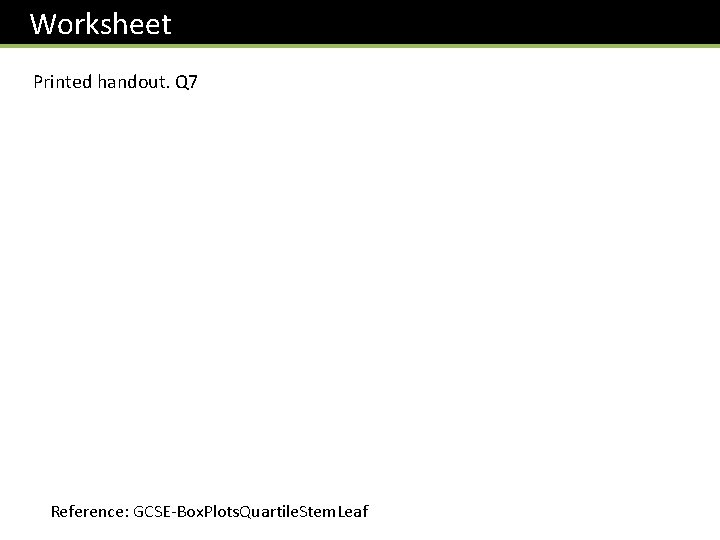Worksheet Printed handout. Q 7 Reference: GCSE-Box. Plots. Quartile. Stem. LeafStem and Leaf ? ? 3 3 7 8 4 1 4 6 5 1 1 2 4 ? 5 5 6 0 2 3 ? Median/ Quartiles? ? ? 1|3 means 13 ?Worksheet Printed handout. Q 3 Reference: GCSE-Box. Plots. Quartile. Stem. LeafRecap: Frequency Tables (f) (x) How would we usually calculate the mean from a list of items? ? Total of values Mean of a list = Num values ? ? = ? ?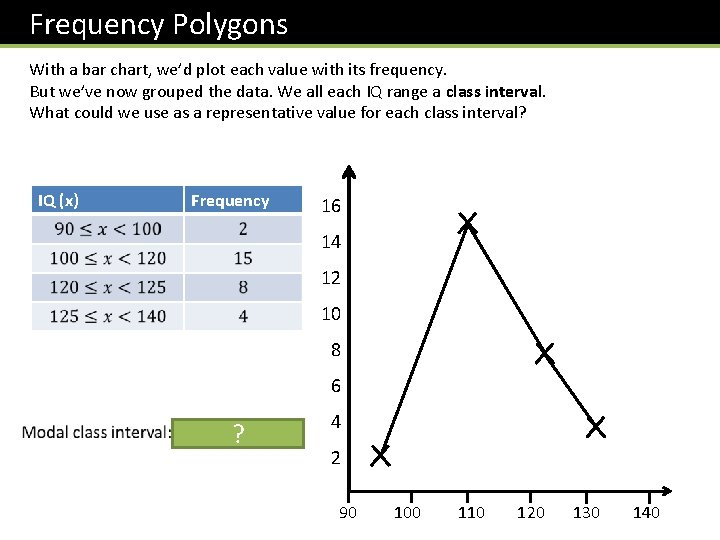Frequency Polygons With a bar chart, we’d plot each value with its frequency. But we’ve now grouped the data. We all each IQ range a class interval. What could we use as a representative value for each class interval? IQ (x) Frequency 16 14 12 10 8 6 ? 4 2 90 100 110 120 130 140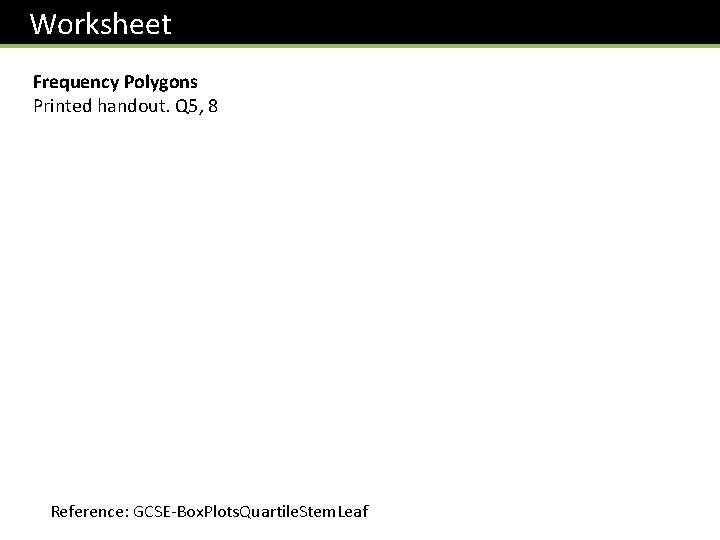Worksheet Frequency Polygons Printed handout. Q 5, 8 Reference: GCSE-Box. Plots. Quartile. Stem. LeafRecap: Grouped Frequency Tables ? Question: Why is our mean going to be an estimate? Because we don’t know the exact times within each range. ?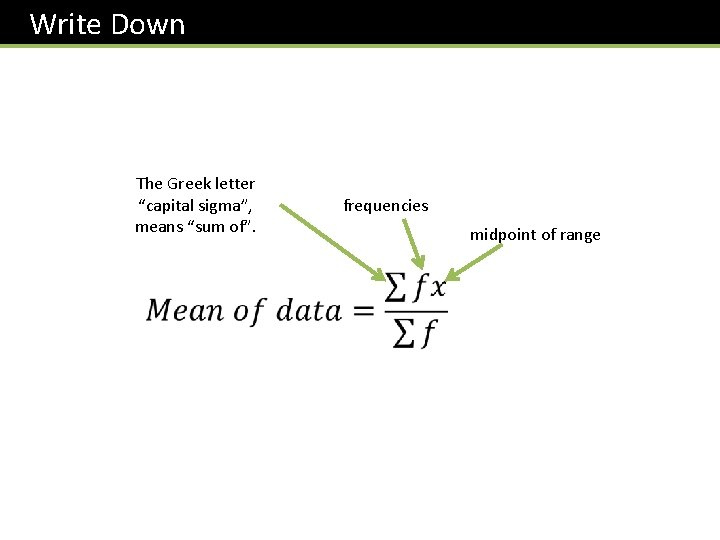Write Down The Greek letter “capital sigma”, means “sum of”. frequencies midpoint of range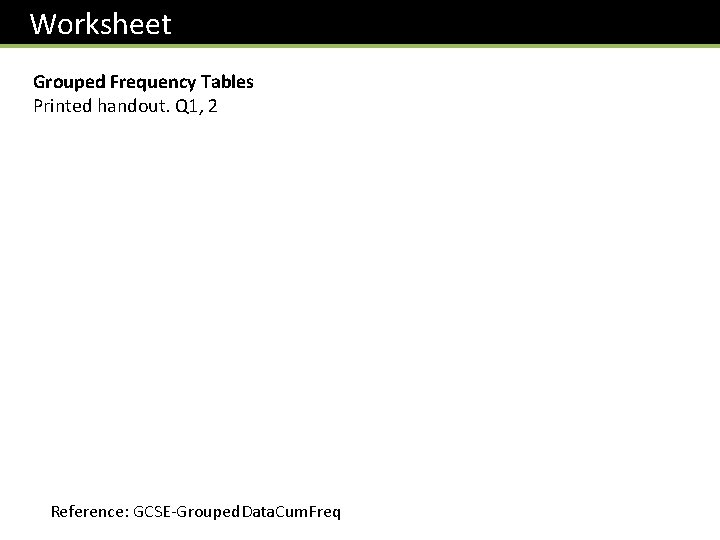Worksheet Grouped Frequency Tables Printed handout. Q 1, 2 Reference: GCSE-Grouped. Data. Cum. FreqMedian from Grouped Frequency Tables IQ (x) Frequency ? ? ? We will see soon that we can actually estimate a value (rather than just give a range) for the median, using something called a cumulative frequency graph.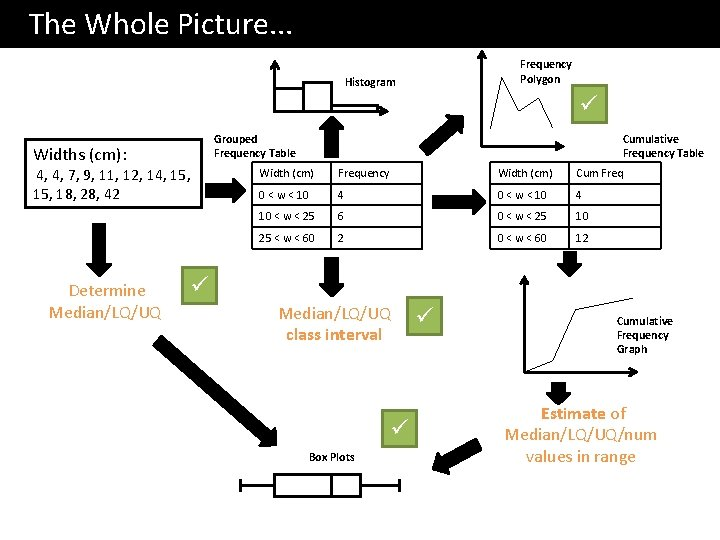The Whole Picture. . . Frequency Polygon Histogram Widths (cm): 4, 4, 7, 9, 11, 12, 14, 15, 18, 28, 42 Determine Median/LQ/UQ Cumulative Frequency Table Grouped Frequency Table Width (cm) Frequency Width (cm) Cum Freq 0 < w < 10 4 10 < w < 25 6 0 < w < 25 10 25 < w < 60 2 0 < w < 60 12 Median/LQ/UQ class interval Box Plots Cumulative Frequency Graph Estimate of Median/LQ/UQ/num values in range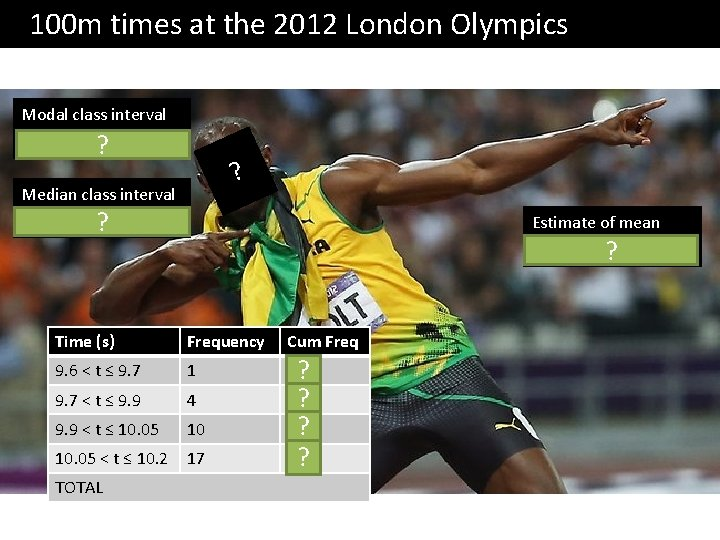100 m times at the 2012 London Olympics Modal class interval 10. 05 < t ≤ 10. 2 ? ? Median class interval 10. 05 < t ≤ 10. 2 ? Estimate of mean 10. 02 Time (s) Frequency 9. 6 < t ≤ 9. 7 1 9. 7 < t ≤ 9. 9 4 9. 9 < t ≤ 10. 05 10 10. 05 < t ≤ 10. 2 17 TOTAL Cum Freq 1? 5? 15? 32? ?32 Cumulative Frequency 28 24 20 16 12 8 4 Time (s) Frequency Cum Freq 9. 6 < t ≤ 9. 7 1 1 Plot 9. 7 < t ≤ 9. 9 4 5 Plot 9. 9 < t ≤ 10. 05 10 15 Plot 10. 05 < t ≤ 10. 2 17 32 Plot Cumulative Frequency Graphs This graph tells us how many people had “this value or less”. Median = 10. 07 s ? Lower Quartile ? = 9. 95 s Upper Quartile ? = 10. 13 s Interquartile Range ? = 0. 18 s 0 9. 5 9. 6 9. 7 9. 8 9. 9 10. 0 10. 1 10. 2 10. 3 Time (s)A Cumulative Frequency Graph is very useful for finding the number of values greater/smaller than some value, or within a range. 32 Cumulative Frequency 28 24 20 16 12 8 4 Cumulative Frequency Graphs Estimate how many runners had a time less than 10. 15 s. 26 ? runners Estimate how many runners had a time more than 9. 95 32 – ? 8 = 24 runners Estimate how many runners had a time between 9. 8 s and 10 s 11 –? 3 = 8 runners 0 9. 5 9. 6 9. 7 9. 8 9. 9 10. 0 10. 1 10. 2 10. 3 Time (s)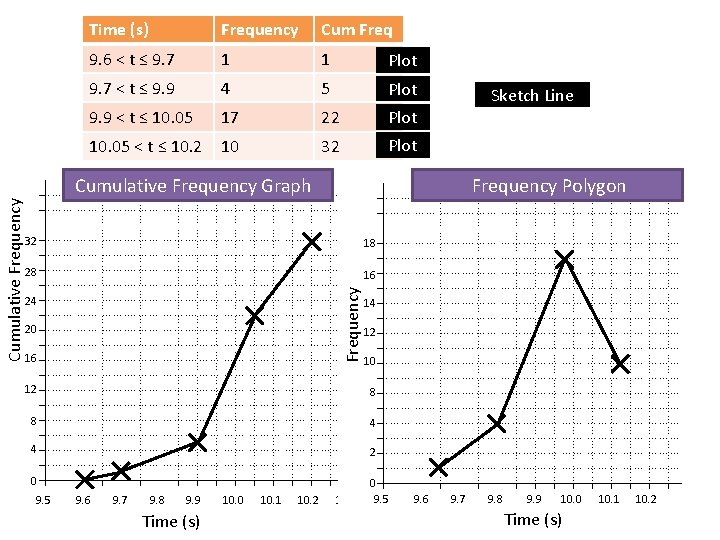Time (s) Frequency Cum Freq 9. 6 < t ≤ 9. 7 1 1 Plot 9. 7 < t ≤ 9. 9 4 5 Plot 9. 9 < t ≤ 10. 05 17 22 Plot 10. 05 < t ≤ 10. 2 10 32 Plot Cumulative Frequency Graph Frequency Polygon 18 28 16 Frequency 32 24 20 16 Sketch Line 14 12 10 12 8 8 4 4 2 0 0 9. 5 9. 6 9. 7 9. 8 9. 9 10. 0 10. 1 10. 2 9. 5 9. 6 9. 7 9. 8 9. 9 10. 0 10. 1 10. 2 10. 3 Time (s)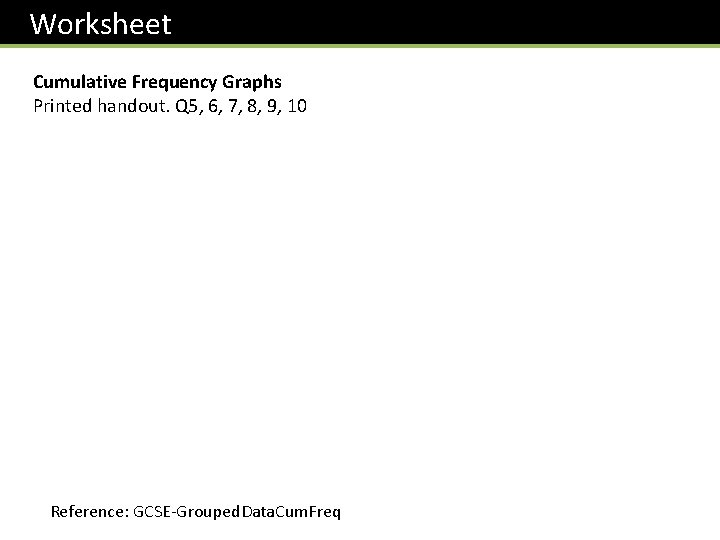Worksheet Cumulative Frequency Graphs Printed handout. Q 5, 6, 7, 8, 9, 10 Reference: GCSE-Grouped. Data. Cum. Freq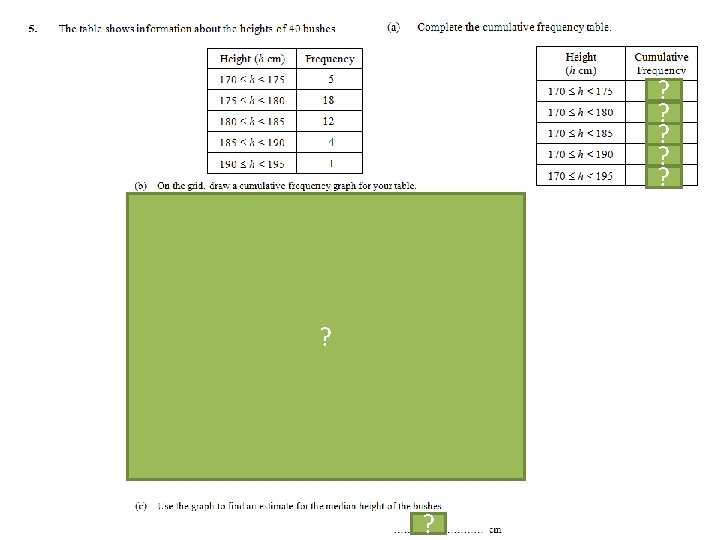5? 23 ? 35 ? 39 ? 40 ? ? 179 ?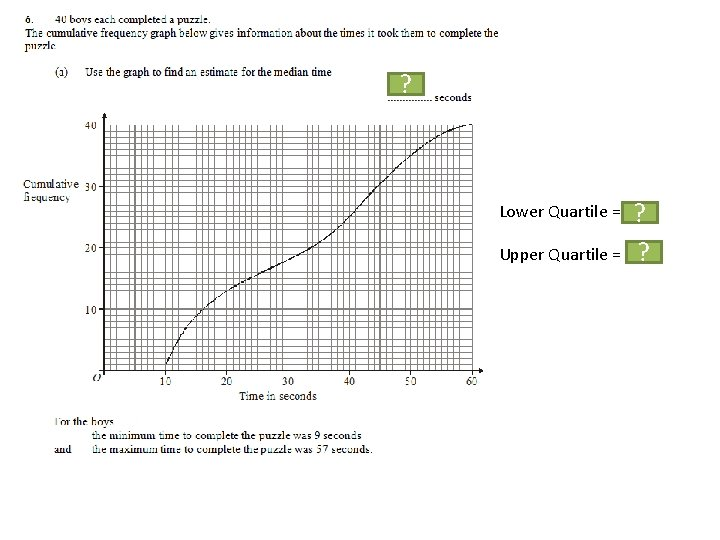34 ? Lower Quartile = 16 ? Upper Quartile = 44. 5 ?We previously found: Minimum = 9, Maximum = 57, LQ = 16, Median = 34, UQ = 44. 5 ? 1 mark: Range/interquartile range of boys’ times is greater. 1 mark: Median of boys’ times is greater. ?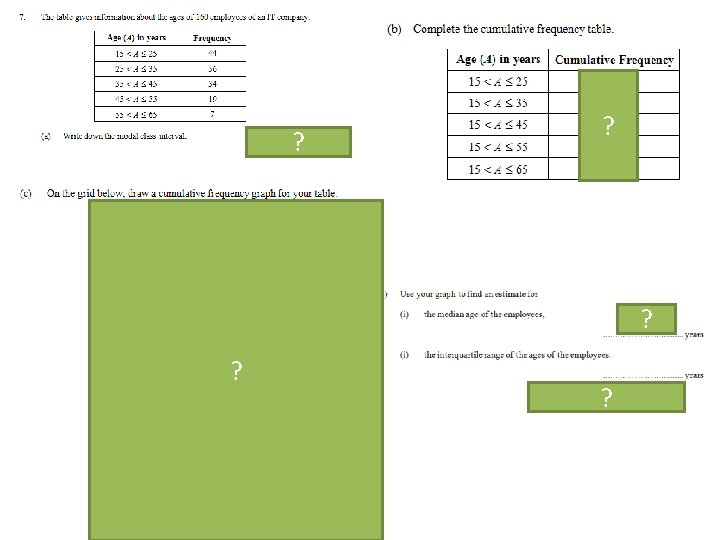44 100 134 153 160 ? ? 30 ? ? ?C? D? B? A?Summary You use a time machine (you made in DT) to travel forward time to when you’re doing your GCSE, in order to give yourself 2 things not to forget when drawing a cumulative frequency diagram. What do you tell yourself? Plot the point with 0 cumulative frequency at the ? START of your first range, not necessarily at the start of the x-axis. Use the END of each range when plotting points, ? so that your cumulative frequency includes all the people in the range.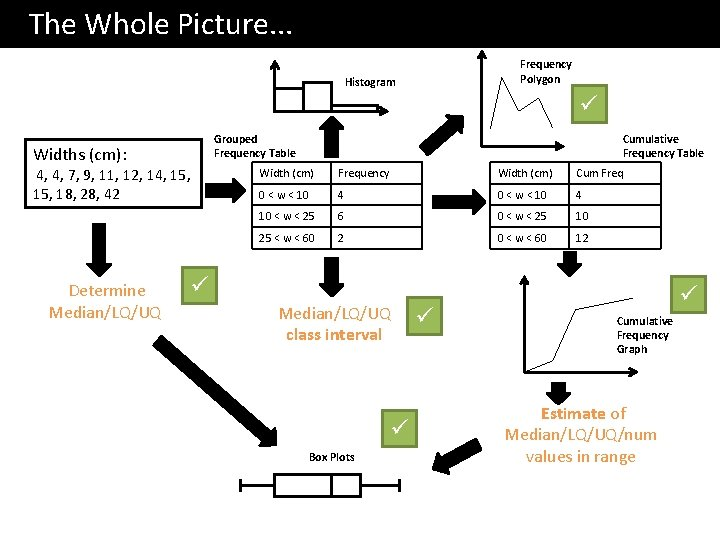The Whole Picture. . . Frequency Polygon Histogram Widths (cm): 4, 4, 7, 9, 11, 12, 14, 15, 18, 28, 42 Determine Median/LQ/UQ Cumulative Frequency Table Grouped Frequency Table Width (cm) Frequency Width (cm) Cum Freq 0 < w < 10 4 10 < w < 25 6 0 < w < 25 10 25 < w < 60 2 0 < w < 60 12 Median/LQ/UQ class interval Box Plots Cumulative Frequency Graph Estimate of Median/LQ/UQ/num values in range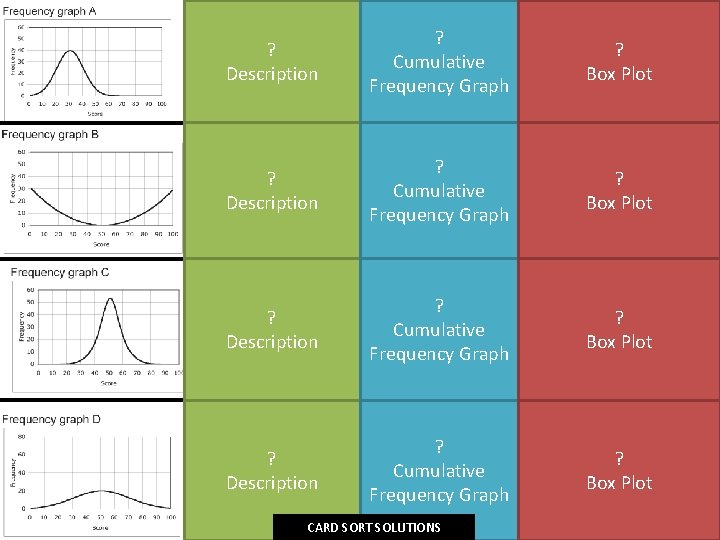? Description ? Cumulative Frequency Graph ? Box Plot CARD SORT SOLUTIONS? Description ? Cumulative Frequency Graph ? Box Plot